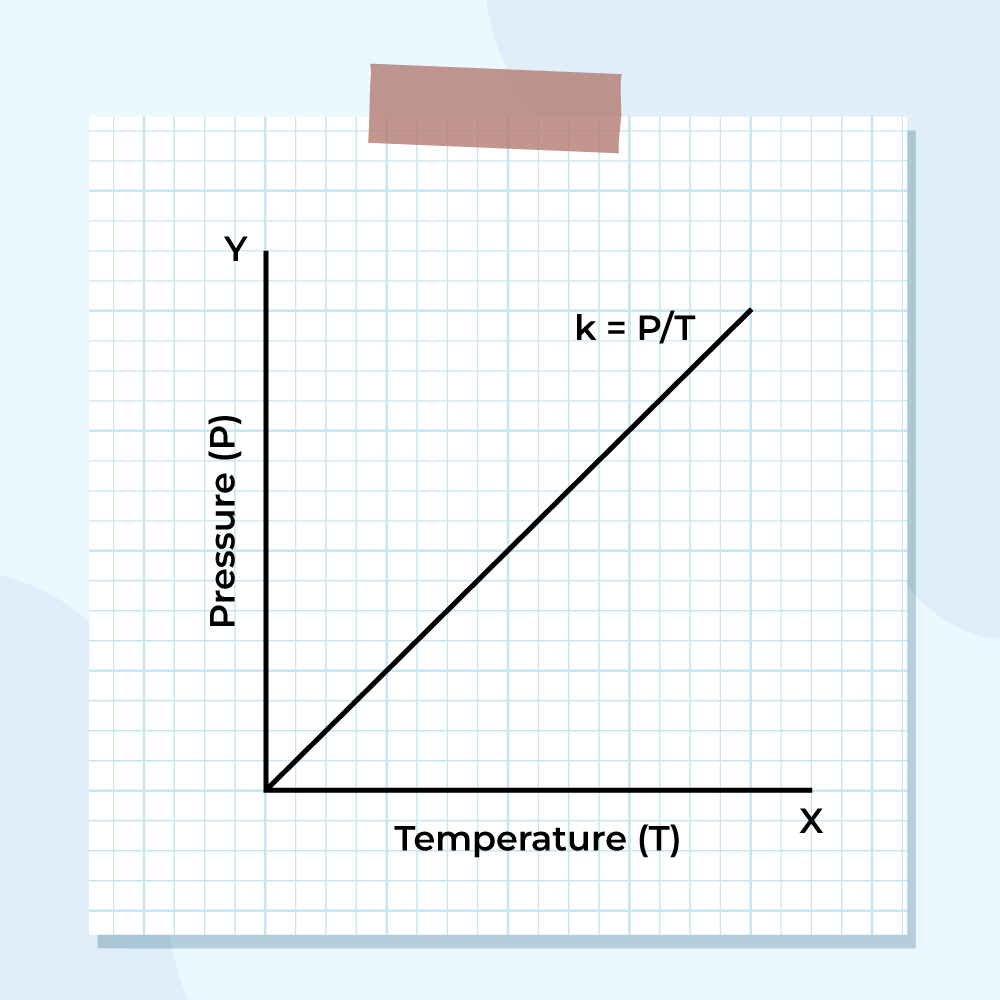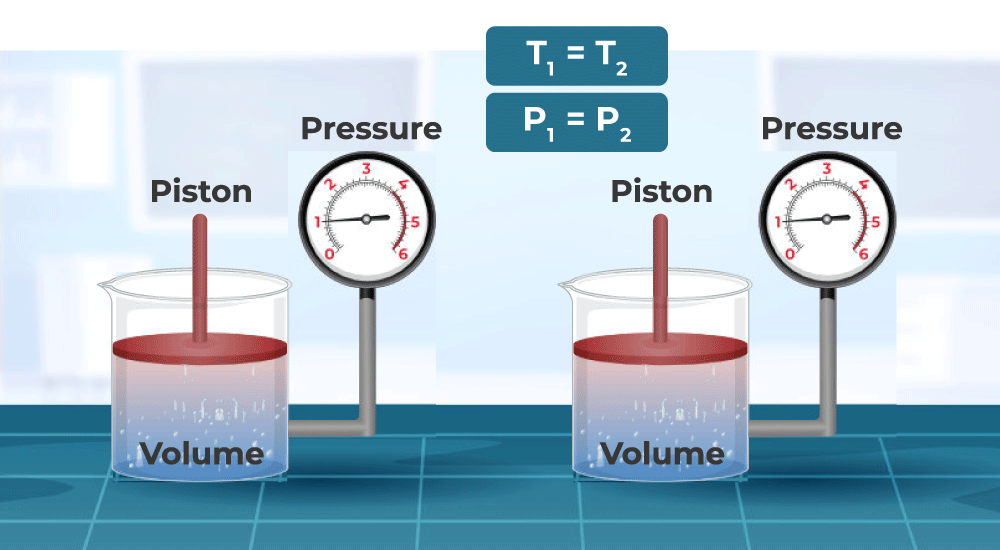GeeksforGeeks App
Open AppBrowser
Continue

# Gay Lussac’s Law

Gay Lussac’s is one of the Ideal gas laws that relates the pressure of the gas with its absolute temperature when its volume is kept constant. The basic statement of Gay Lussac’s Law is, the pressure produced by a gas is directly proportional to its temperature if mass and volume are kept fixed. Gay Lussac’s Law has wide application in sciences and also in our everyday life. Gay Lussac’s Law as the name suggests was given by the French chemist Joseph Gay-Lussac in the year 1808.

## What is Gay-Lussac’s Law?

Gay-Lussac’s law states that the pressure exerted by a gas varies directly with the absolute temperature of the gas if the mass of the gas is fixed and the volume is constant. i.e. The pressure exerted by a gas is proportional to the temperature of the gas at constant volume.

P ∝ T

P = kT

Now, at temperature T1 and pressure P1

P1 = kT1…(1)

Also, at temperature T2 and pressure P2

P2 = kT2…(2)

Now, combining eq(1) and eq(2)

P1 / T1 = P2 / T2

P is the pressure exerted by the gas
T is the absolute temperature of the gas

## Gay Lussac’s Law Graph

Gay Lussac’s law is expressed by the equation,

P = kT…(1)

Mathematically this equation is comparable to y = mx…(2)

Comparing eq (1) with eq (2) we get

y = P, m = k and x = T. Now the graph of the given equation is,## Formula and Derivation

The formula for Gay-Lussac’s Law is,

P1/T1 = P2/T2

where,
P1 is the initial pressure,
T1 is the initial temperature,
P2 is the final pressure,
T2 is the final temperature

### Derivation of Gay Lussac’s Law

It is known that the pressure of a gas is proportional to its temperature. This relation can be written as,

P ∝ T

Replacing the proportionality sign with constant k we get,

P = kt

The above equation is valid for all values of pressure and temperature.

Suppose a gas is exerting pressure P1 at a temperature T1. The equation that defines this situation is,

P1 = kT1

k = P1/T1……… (1)

The temperature of the gas changes to T2, which changes the pressure to P2. This can be expressed as,

P2 = kT2

k = P2/T2……… (2)From (1) and (2) we get,

P1/T1 = P2/T2

This derives the Gay Lussac law for gases.

## Gay Lussac’s Law of Gaseous Volumes

Joseph Louis Gay-Lussac in 1808 also proposed the law of Gaseous Volumes which is different from the law of definite proportions. According to Gay Lussac’s Law of Gaseous Volumes, if measured at the same temperature and pressure, the ratio of the volumes of reacting gases are small whole numbers. Gay Lussac’s Law of Gaseous Volumes works with respect to volume while the law of definite proportion works with the mass of the gas.

## Examples of Gay Lussac’s Law

Gay Lussac’s Law has a variety of applications some of the examples of Gay Lussac’s law that we observed in our daily life are,

• Increasing the heat to a pressure cooker increases the pressure inside it. This results in less cooking time as the steam does not lose the taste of food to the air due to the sealed container.
• If too much air is put in vehicle tyres, they may over-pressurize due to high temperature. But on a cold day, their pressure reduces which results in underinflation.
• Aerosol cans shouldn’t be kept in high temperatures because heat raises the pressure inside them which may lead to an explosion.

## Solved Examples on Gay Lussac’s Law

Example 1: The initial pressure of a gas inside a tank is 2 Pa at 100 K. Find the final pressure if the gas is heated to a temperature of 200K.

Solution:

We have,

Initial Pressure P1 = 2 Pa
Final Pressure P2 = ?
Initial Temperature T1 = 100 K
Finial Temperature T2 = 200 K

Using the formula we get,

P1/T1 = P2/T2

2/100 = P2/200

P2 = 200/50

P2 = 4 Pa

Final pressure of the gas P2 = 4 Pa

Example 2: The final pressure of a gas inside a tank is 12 Pa at 200 K. Find the initial pressure if the initial temperature of the gas was 50 K.

Solution:

We have,

Initial Pressure P1 = ?
Final Pressure P2 = 12 Pa
Initial Temperature T1 = 50 K
Finial Temperature T2 = 200 K

Using the formula we get,

P1/T1 = P2/T2

P1/50 = 12/200

P1/50 = 3/50

P1 = 3 Pa

Initial Pressure of the gas P1 = 3 Pa

Example 3: The initial pressure of a gas inside a tank is 2 Pa at 300 K. Find the final temperature of gas which increased its pressure value to 8 Pa.

Solution:

We have,

Initial Pressure P1 = 2 Pa
Final Pressure P2 = 8 Pa
Initial Temperature T1 = 300 K
Finial Temperature T2 = ?

Using the formula we get,

P1/T1 = P2/T2

2/300 = 8/T2

T2/8 = 300/2

T2/8 = 150

T2 = 1200 K

Finial Temperature of the gas T2 = 1200 K

Example 4: The final pressure of a gas inside a tank is 15 Pa at 900 K. Find the initial temperature if the initial pressure of gas was 3 Pa.

Solution:

We have,

Initial Pressure P1 = 15 Pa
Final Pressure P2 = 3 Pa
Initial Temperature T1 = ?
Finial Temperature T2 = 900 K

Using the formula we get,

P1/T1 = P2/T2

3/T1 = 15/900

T1/3 = 900/15

T1/3 = 60

T1 = 180 K

Initial Temperature of the gas T1 = 180 K

## FAQs on Gay-Lussac’s Law

### Q1: What is Gay Lussac’s law formula?

Gay Lussac’s law is an Ideal gas law that deals with the ideal gas at constant volume. According to this law, at constant volume, the pressure of the gas is directly proportional to the temperature of the gas. Gay Lussac’s law formula is,

P / T = constant

### Q2: What does Charles law state?

According to Charles law, “At constant pressure, the volume of an ideal gas is directly proportional to its absolute temperature.”

### Q3: Why is Gay Lussac’s law important?

Gay Lussac’s law is important because, with its help, we can find the temperature of the gas if the pressure variation of the gas is given at constant volume.

### Q4: What are the types of Ideal Gas Laws?

There are four different types of Ideal Gas Laws, which are

• Boyle’s Law
• Charles’s Law
• Gay Lussac’s Law

### Q5: What is Avogadro’s law?

According to Avogadro, “The number of molecules of different gases at similar conditions of temperature, pressure, and volume is always the same.”